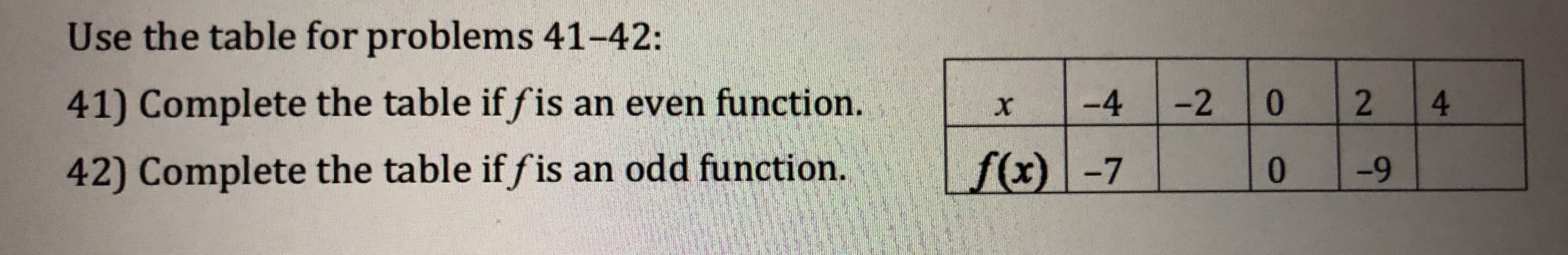# Use the table for problems 41-42:41) Complete the table if fis an even function.42) Complete the table if fis an odd function. f(x) -70r4-2 0 2 4-9

Question
2 viewshelp_outlineImage TranscriptioncloseUse the table for problems 41-42: 41) Complete the table if fis an even function. 42) Complete the table if fis an odd function. f(x) -70 r4-2 0 2 4 -9 fullscreen
check_circle

Step 1

a) A function f(x) is said to be  even, if f(-x)=f(x), using this we can calculate f(-2) and f(4) as shown on board.

Step 2

b) A function f(x) is said to be  odd, if f(-x)=-f(x), usin...

### Want to see the full answer?

See Solution

#### Want to see this answer and more?

Solutions are written by subject experts who are available 24/7. Questions are typically answered within 1 hour.*

See Solution
*Response times may vary by subject and question.
Tagged in

### Algebra